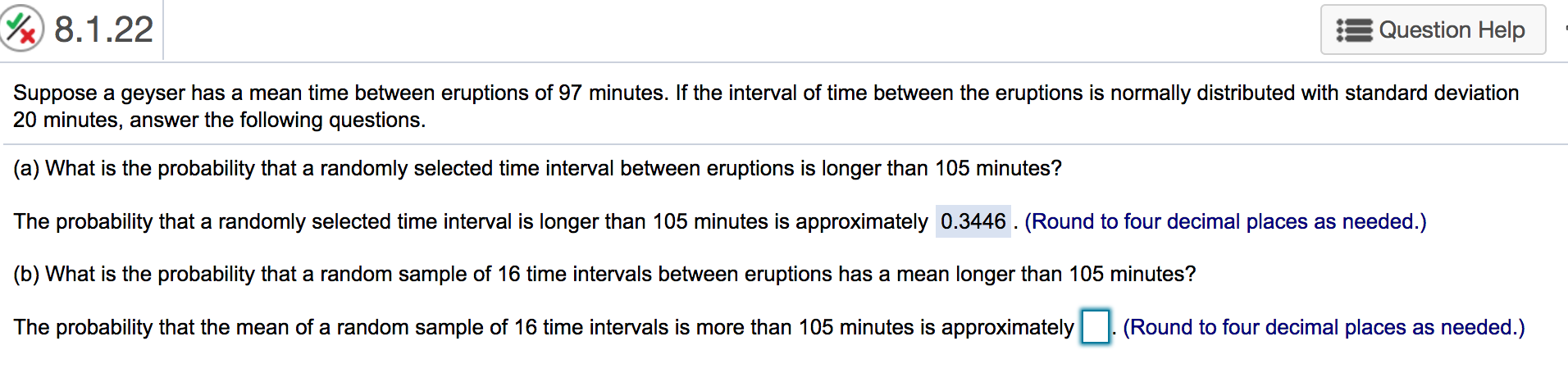# 8.1.22Question HelpSuppose a geyser has a mean time between eruptions of 97 minutes. If the interval of time between the eruptions is normally distributed with standard deviation20 minutes, answer the following questions.(a) What is the probability that a randomly selected time interval between eruptions is longer than 105 minutes?The probability that a randomly selected time interval is longer than 105 minutes is approximately 0.3446. (Round to four decimal places as needed.)(b) What is the probability that a random sample of 16 time intervals between eruptions has a mean longer than 105 minutes?(Round to four decimal places as needed.)The probability that the mean of a random sample of 16 time intervals is more than 105 minutes is approximately

Question
23 views

I'm lost..help_outlineImage Transcriptionclose8.1.22 Question Help Suppose a geyser has a mean time between eruptions of 97 minutes. If the interval of time between the eruptions is normally distributed with standard deviation 20 minutes, answer the following questions. (a) What is the probability that a randomly selected time interval between eruptions is longer than 105 minutes? The probability that a randomly selected time interval is longer than 105 minutes is approximately 0.3446. (Round to four decimal places as needed.) (b) What is the probability that a random sample of 16 time intervals between eruptions has a mean longer than 105 minutes? (Round to four decimal places as needed.) The probability that the mean of a random sample of 16 time intervals is more than 105 minutes is approximately fullscreen
check_circle

Step 1

From the given information, mean (μ) = 97 minutes and standard deviation (σ) = 20minutes.

Let ‘X’ be a normal distribution random variable.

Step 2

(a) The probability that a randomly selected time interval between eruptions is longer than 105 minutes is,

Step 3

Therefore, the probability that a randomly selected time interva...

### Want to see the full answer?

See Solution

#### Want to see this answer and more?

Solutions are written by subject experts who are available 24/7. Questions are typically answered within 1 hour.*

See Solution
*Response times may vary by subject and question.
Tagged in

### Statistics